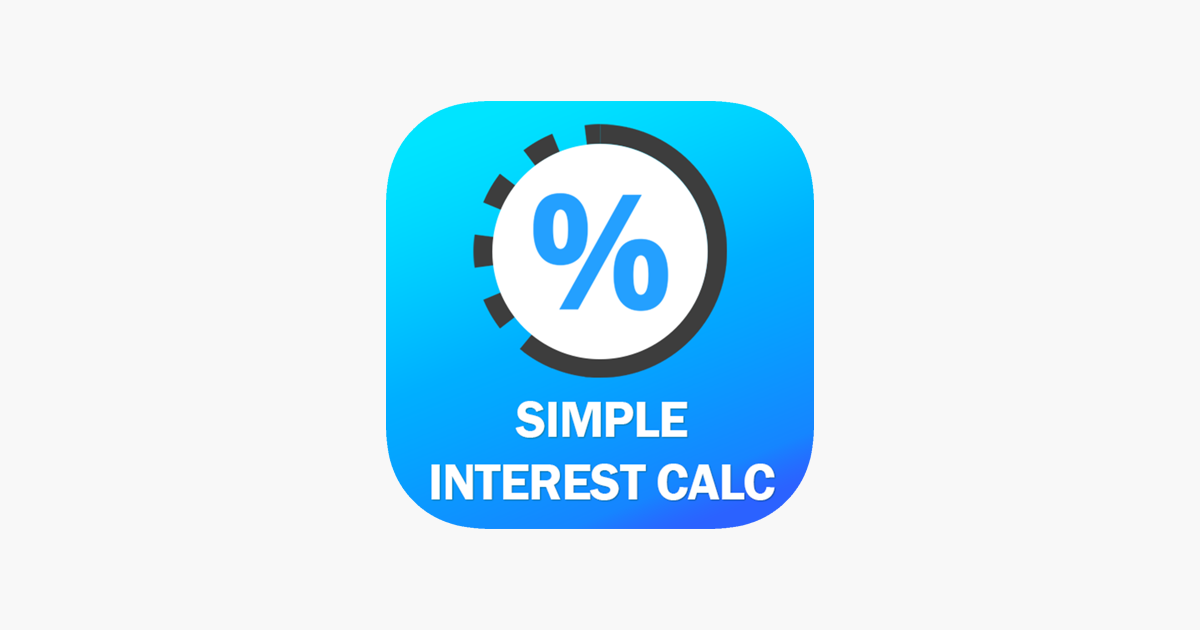# Simple Interest Calculator + – Nirav Patel## Description

== Simple Interest Calculator ==

Simple interest calculator calculates interest of any amount on any interest rate fast, accurate & easily. you can calculate your interest on monthly or yearly interest rate.

You can easily calculate interest between any two date by just providing start date and end date. if you have to calculate interest of any specific duration then you can do it here by giving duration in years month or days.

Anyone can use this interest calculator easily and accurately.